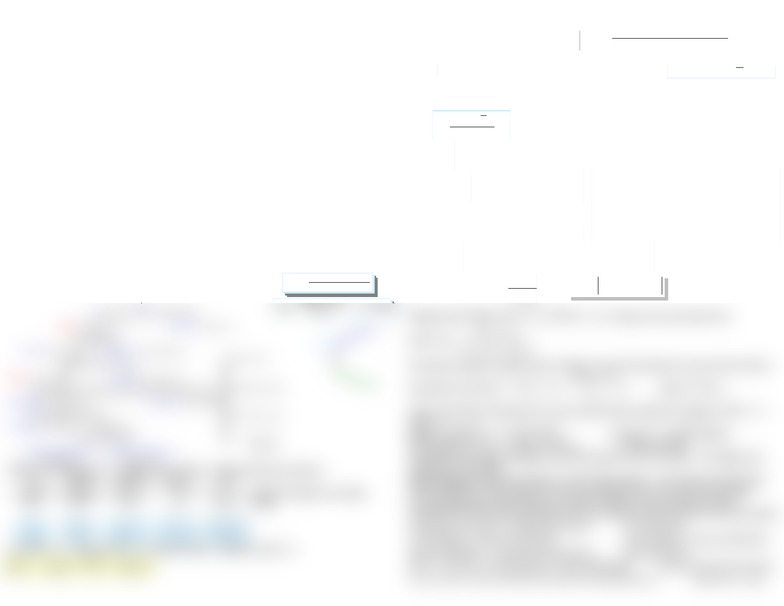Study Guides (390,000)
CA (150,000)
WLU (6,000)
BU (1,000)
BU473 (4)

# BU473 Study Guide - X86 Instruction Listings, Liquigas, Automobilclub Von Deutschland

Department
Course Code
BU473
Professor
Ning Tang

This preview shows half of the first page. to view the full 1 pages of the document.Share Price
EPS:profits/Shares ×P/E ratio: Price/EPs
ROE: Profit/Equity Book Value: Equity/Shares:×
ROA:Profits/Assets Leverage:Assets/Equity×
Profitability: Profits/Sales Turnover: Sales/Assets×
Coverage: Profits/EBIT EBIT/Sales
×
Operating Efficiency
EBIT/Margin
Production Efficiency
Margin/Sale
×
Sales/Cash
Sales/Receivable
Sales/Inventory
Sales/Fixed
Assets
Eqty
TA
TA
Sales
Sales
EBIT
EBIT
EBT
EBT
NI
Tax
Burden Interest
Burden EBIT
Efficiency TA
Turnover Leverage
Ratio
EQUITY
TA
TA
Sales
SALES
NI
Equity
NI
Net Profit
Margin Asset
Turnover Leverage
Ratio
B
BEt P)P(PCF
TR
B
BEt P)P(PCF
TR
RR  
CF P
P
t E
B1 TR
)
n
TR1)...(
2
TR1)(
1
TR1(
0
WI
CPC
CWI
YI
YI
CWI
CPC
1
For.Curr. of Val.Begin For.Curr. of Val.End
RR
1)TR1)...(TR1)(TR1( n/1
n21
  sX1G1 2
2
2
 
s X X
n 1
21/2
]))([(
1
22 i
m
iiprRER
n
iiip REwRE
1
)()(
)()()( BBAAp REwREwRE
BABABBAAP wwww ,
22222 2
 
 
 
m
i
m
ji
i
m
jijjiii
m
i
m
jijjiP
www
ww
1 1 1
22
1 1
2
m
iiBiBAiABA prRERRER )]()][([ ,,,
BA
BA
,
BA,
p
s
fs
fp
RRE
RRE
)(
)(
2
5.0)(
AREEU
BBAAP ww
Bottom-up approach: firm-specific, work upwards Put Option: rgt to Sell
Top-down approach: analyze economy, then industry, then firm
CAPM: Rf = real RoR + inf…. k = Rf + Rp Fisher eq’n: 1+i = (1+r)(1+inf)
effct mkt take passive approach b/c no active strat beat it on risk-adjusted
value of analyst report depends on: amt of recent info, # of anlyts follwng stock,
degree of consensus, quality of analysts.
Defensive stocks unhurt by bus. cycle downside…TAA: tactical asset allocation
takes advantage of mkt timing skills (ST dev from LT asst mixes)
Technical Analysis: unconcerned w/ underlying econ vars affting firms/mkt
GDP = G+C + I + (X M) = Gov’t Spnd + Consmr Spend + bus Invst + (Cdn Ex Im)
Normal unemplymt frictional; structural unemp natural umemp rate; cyclical
Bus Cyc: expansion = durabls, peak=nat resurce+energy, contrctin=defensive ind,
trough=cap gds…”soft landing”=econ growth slows but not neg…leading ind:
stocks,money supp, wrk week, sales of consumer durables…extreme times, econ
Coincident + lagging indicators…interest rate determinants: inf rate, xchg rate,
gov’t deficits, BoC, default risk of the borrower…
CERI =Cdn effect. xchg rte index is trade-weighted msre of xchg rte
P/E tends to b high whn inf and int rates are low
NAICS=NA Indust Classif Syst, SIC=Std Indus clas11divs, lgr digits, more spfc
Pionerng stg(most risk), expns stg (hi invmt funds, strt divs), stablztn (avd if desire
cap gains), dclng (new prds, low ror)
4 crucial factors asing ind: Historicals, compttin, gov’t, strctl chng
Cyclical ind=most affctd by recessn
CICA and FASB create acctng stdrds in CA and USA
P0/E1 = (D1/E1)/(k-g)…k = firm’s bond yield + equity-bond risk premium
=ROA*TA/eqtyLeverage
=ROE
g = ROE * (1 payout ratio)(1-payout ratio) = retention ratio = b
Cum Wealth Ind CWIn=
TRn = (CWIn/CWIn-1) - 1
Int’l Rtrns: TR in domestic currency =
TR, RR, CWI only good for single time period…
For several periods use arithmetic or geometric means
Geo = diff b/w geo & arith:
Adjust for inf: TRia = [(1+TR)/(1+CPI)] 1 OR CWIia = CWI/CIinf
CI = (1 + GM of inflation rate)^n
Sources of Risk: Int, Mkt, Inf, Busi, Finan, Liq, Xchg, Country
Std Dev “Equity Risk Prem” and “Bond Default Prem
ERP=[(1+TR)/(1+RF)] 1
Variance:
n-asset case:
2-asset case:
Covariance: i
Corr Coeff:
Single-Index Model: TRit = αi + βi TRMt + εit unique risk and market risk
MVP: Wa =
BABA
BAB
,
22
,
2
2
Port theory asptns: single invt per, liqdty, inv pref only based on exp risk and return
Cap Alloc Line (CAL): slope: 2nd term
CAL w/ leverage: proportion to be borrowed at Rf is stated as negative; wRF + (1
wRF) = 1
E(Rp) = wRF(RF) + (1-wRF)*E(Rt) std devp = (1-wRF)*stddevt
Exp Utility Fcn: find the sub/pd
The greater the slope of indiff curve, the greater the risk aversion…the higher the
indiff curve, the better
Sep Theorem: tailoring portfolio to client inappropriate all investors should hold
same portfolio of risky assets & move up and down the risk-return line through
borrowing/lending the decision of which portfolio of risky assets to hold is
separate from the financing decision (how to allocate funds b/w RF and risky assets)
Total Asset Turnover = Sales/Total Assets Lev=Assets/SE
Profit Margin = Net Income/Sales Gross Margin = gross profit/sales
Equity Multiplier = Total Asts/Total Equity ROA=NI/Assets
ROE = Turnover x Profit Margin x Equity Multiplier Quick ratio=quick assts/CL
inv TO ratio = total COGS/avg inventory ROS=EBIT/sales NAV=asts liab